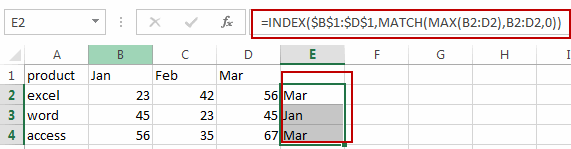How to Extract the Column Header of the Largest Value in a Row in Excel

This post will guide you how to find the largest value in a row and return column header in Excel. How to retrieve the column header of the largest value in a row with a formula in Excel. How to return the column title of the max value in the row in Excel.

Return the Column Header of the Largest Value in a Row

Assuming that you have a list of data in range A1:D4, in which contain sales data for each month. And you want to find the highest value for each product and return the Month column header in your worksheet. How to do it. You can use an Excel formula based on the INDEX function, the MATCH function and the MAX function. Like this:

=INDEX(\$B\$1:\$D\$1,MATCH(MAX(B2:D2),B2:D2,0))

You need to type this formula into cell E2 and press Enter key on your keyboard, and then copying this formula from cell E2 to range E3:E4, you would notice that you will get the column header for in every row.Let’s see how this formula works:

The INDEX function will return a reference of the cell at the intersection of a specific row and column in range B1:D1.

The MAX function will return the maximum number from a given range B2:D2. And pass the returned result into the MATCH function as its argument.

The MATCH function will return the relative position of the maximum value in the given range.

Related Functions

• Excel INDEX function
The Excel INDEX function returns a value from a table based on the index (row number and column number)The INDEX function is a build-in function in Microsoft Excel and it is categorized as a Lookup and Reference Function.The syntax of the INDEX function is as below:= INDEX (array, row_num,[column_num])…
• Excel MATCH  function
The Excel MATCH function search a value in an array and returns the position of that item.The MATCH function is a build-in function in Microsoft Excel and it is categorized as a Lookup and Reference Function.The syntax of the MATCH function is as below:= MATCH  (lookup_value, lookup_array, [match_type])….
• Excel MAX function
The Excel MAX function returns the largest numeric value from the numbers that you provided. Or returns the largest value in the array.= MAX(num1,[num2,…numn])…
Related Posts

Extract all the matches with helper Column

With Excel's powerful functions IF, INDEX, and MATCH, we can find exactly what you're looking for with a few clicks of the mouse. This step-by-step tutorial will show how easy it is to extract data using these tools and more! ...

How to Find the Earliest and Latest Date in Excel

We have a range of dates and we want to look up the earliest and the latest date based on certain criteria like the earliest date for a showing movie, we can use MIN and MAX functions with IF function ...

How to Create Dynamic Drop Down List without Blank in Excel

This post will guide you how to create dynamic drop down list without blank cells in Microsoft Excel. In Excel, and you can use Data Validation feature to improve the efficiency of data entry in excel, and it also be ...

How to Calculate the Average Excluding the Smallest & Highest Numbers in Excel

Calculating the average for a batch of data is frequently used in our daily life. But for some cases like statistic the average score in a competition, or price analysis, we often calculate the average excluding the smallest and highest ...

How to Get the Maximum or Minimum Absolute Value in Excel

It is easy to find the maximum or minimum value in a batch of data in excel, but if this batch of numbers contains both positive and negative numbers, the maximum or minimum absolute value cannot be found out by ...

How to Find the Largest Value in a Column and Return the Adjacent Cell Value in Excel

This post will guide you how to find the largest value in a column and then return the adjacent cell value with a formula in Excel. How do I find the max value and return the cell value from the ...

How to Find Max And Min Value with Single or Multiple Criteria in Excel

This post will guide you how to find the maximum value in a range of cells based on single or multiple criteria in Excel. How do I calculate the Minimum value based on criteria with MIN Function in Excel. Find ...

How to Check If value Is between Two Numbers in Excel

This post will guide you how to check if a cell value is between two numbers in Excel. How do I build an IF statement that test if a cell value is between two given values in formula in Excel. ...

How to Get Cell Address of Max or Min Value in a Range in Excel

This post will guide you how to return the cell address of a maximum cell value from a given range of cells with a formula in Excel. How do I Find the minimum value from a given range and return ...

How to Highlight The Highest and Lowest Value in Each Row or Column in Excel

This post will guide you how to highlight the highest value in each row or column in Excel. How do I highlight the lowest value in each row and column with conditional formatting function. Highlight Highest Value in Each Row ...

Sidebar